# Difference between revisions of "5"

## Summary

### Factorization

The number 5 is a prime number.

### Properties and families

Property or family Parameter values First few numbers Proof of satisfaction/membership/containment
prime number it is the third prime number 2, 3, 5, 7, 11, 13, 17, 19, 23, 29, 31, 37, 41, 43, 47, 53, 59, 61, 67, 71, 73, 79, 83, 89, 97, [SHOW MORE]View list on OEIS divide and check
Fermat number, i.e.,3, 5, 17, 257, 65537, 4294967297 View list on OEIS plug and check
Fermat prime (Fermat number that is also a prime number) combine above
safe prime (prime of the form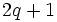,prime) first safe prime 5, 7, 11, 23, 47, 59, 83, 107, 167, [SHOW MORE]View list on OEIS, 2 is prime
Sophie Germain prime (primesuch that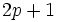is prime third Sophie Germain prime 2, 3, 5, 11, 23, 29, 41, 53, 83, 89, [SHOW MORE]View list on OEIS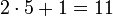, 11 is prime
regular prime second regular prime (2 is neither regular nor irregular) 3, 5, 7, 11, 13, 17, 19, 23, 29, 31, 41, 43, 47, 53, 61, [SHOW MORE]View list on OEIS
twin primes (greater member of pair) first greater member of twin prime pair 5, 7, 13, 19, 31, 43, 61, 73, 103, [SHOW MORE]View list on OEIS 5 - 2 = 3 is a prime
twin primes (lesser member of pair) second lesser member of twin prime pair 3, 5, 11, 17, 29, 41, 59, 71, 101, [SHOW MORE]View list on OEIS 5 + 2 = 7 is a prime
near-square prime of the form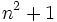(second prime of this form) 2, 5, 17, 37, 101, 197, 257, [SHOW MORE]View list on OEIS

## Structure of integers mod 5

### Discrete logarithm

2 is a primitive root mod 5, so we can take it as the base of the discrete logarithm. We thus get an explicit bijection from the additive group of integers mod 4 to the multiplicative group of nonzero congruence classes mod 5, given by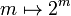. The inverse of that mapping is the discrete logarithm, i.e., the discrete logarithm ofismeans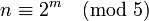:

Congruence class mod 5 (written as smallest positive integer) Congruence class mod 5 (written as smallest magnitude integer) Discrete logarithm to base 2, written as integer mod 4 Is it a primitive root mod 5 (if and only if the discrete log is relatively prime to 4)? Is it s quadratic residue or nonresidue mod 5 (residue if discrete log is even, nonresidue if odd)
1 1 0 No quadratic residue
2 2 1 Yes quadratic nonresidue
3 -2 3 Yes quadratic nonresidue
4 -1 2 No quadratic residue

We could choose an alternative discrete logarithm, where we use 3 as the base. This is simply the negative of the original discrete logarithm:

Congruence class mod 5 (written as smallest positive integer) Congruence class mod 5 (written as smallest magnitude integer) Discrete logarithm to base 3, written as integer mod 4 Is it a primitive root mod 5 (if and only if the discrete log is relatively prime to 4)? Is it s quadratic residue or nonresidue mod 5 (residue if discrete log is even, nonresidue if odd)
1 1 0 No quadratic residue
2 2 3 Yes quadratic nonresidue
3 -2 1 Yes quadratic nonresidue
4 -1 2 No quadratic residue

### Primitive roots

The number of primitive roots equals the number of generators of the additive group of integers modulo 4, which is the Euler totient function of 4, namely 2. Given any primitive root, the primitive roots are preciselyand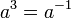.

The explicit list of primitive roots is 2 and 3.

We note the following:

Of the four congruence classes of invertible elements, there are two classes of quadratic residues and two classes of quadratic nonresidues. Given an primitive root, the quadratic residues are 1 andand the quadratic nonresidues areand. Note that it's on account of 5 being a Fermat prime that every quadratic nonresidue is a primitive root. See quadratic nonresidue equals primitive root for Fermat prime.